## 生成完整的配置文件

### 一般做法可以考虑继承base然后一个个实现模块，也可以先整体跑通在一个个修改，一般采用第二种 方法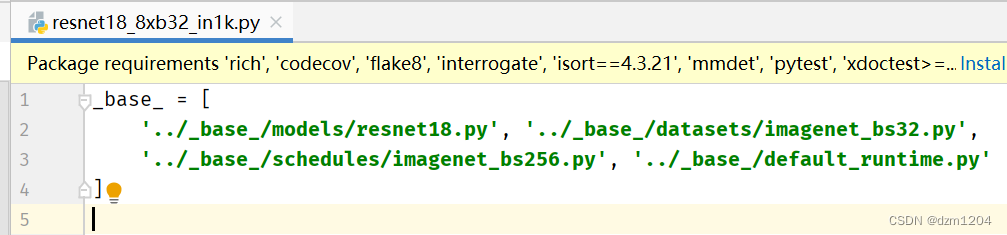### 先复制resnet配置文件的绝对路径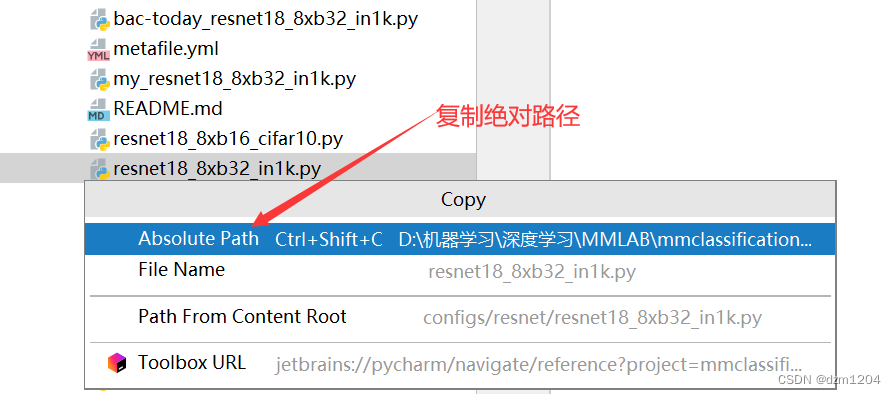### 找到tools下的train.py就是入口函数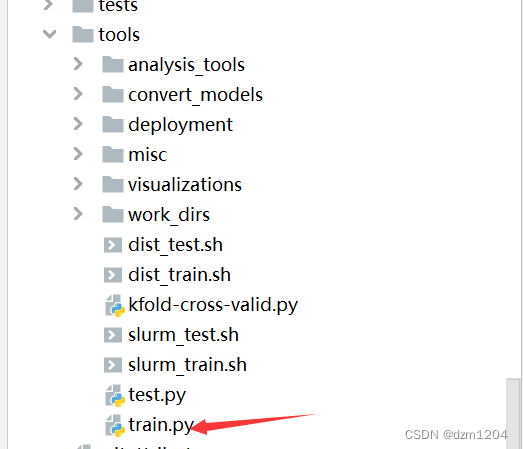### 这里的路径是默认参数，所以直接给路径复制到参数设置里面去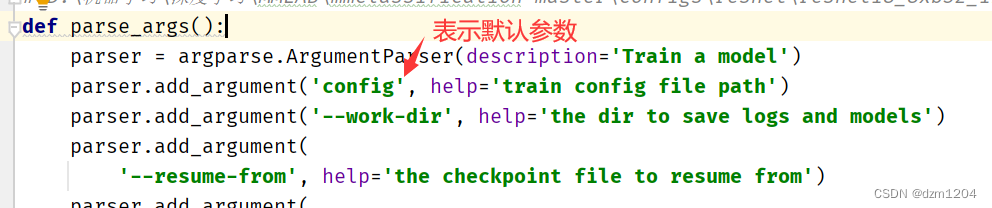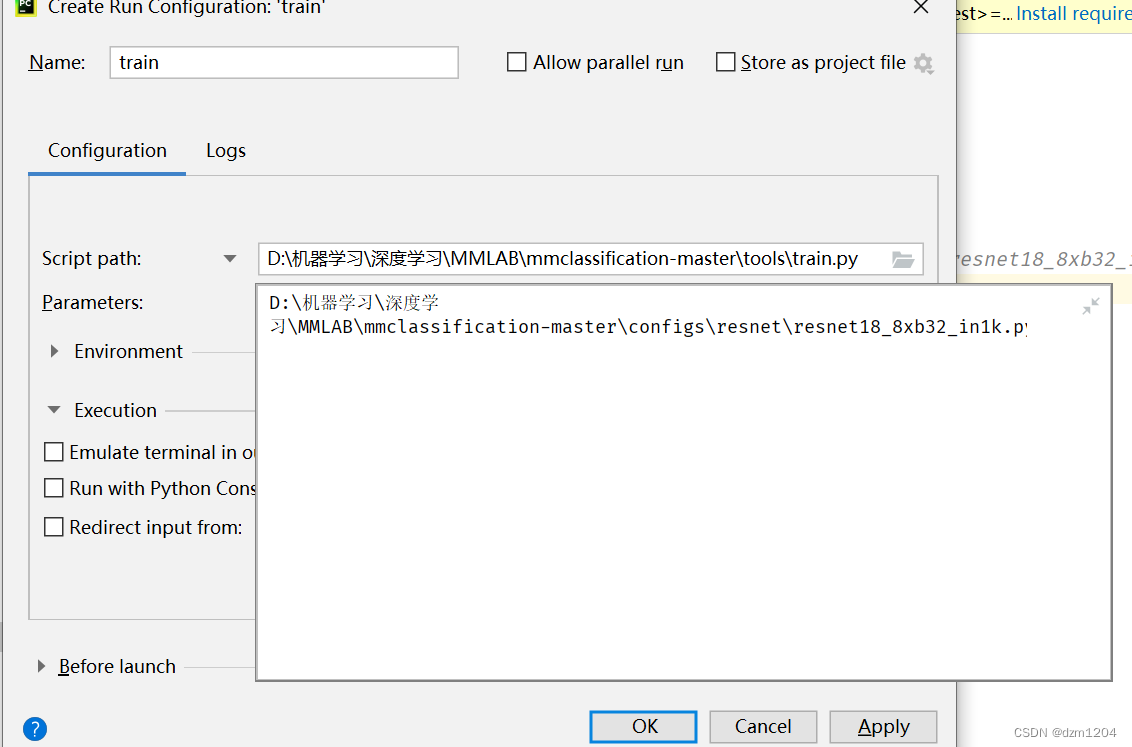### 参数配置完可以先试着跑一下，虽然会报错但是也会生成一个文件如下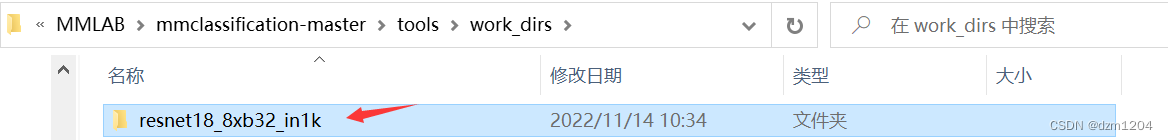### 会生成这个配置文件，接下来就可以直接复制到pycharm中改这个配置文件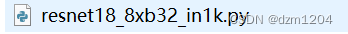### 重命名后，复制到pycharm打开，里面就是生成的自己的网络结构，一开始里面都一些默认配置，我们需要修改一些配置项，最多修改的就是输出大小修改成自己分类的类别数，和指定自己训练集、验证集、测试集的文件路径，工作空间路径以及迭代多少次保存模型和日志文件。修改后一般可以直接跑通

# 数据集存放在MMLAB\mmclassification-master\mmcls\data\flower_data\下
model = dict(
type='ImageClassifier',
backbone=dict(
type='ResNet',
depth=18,
num_stages=4,
out_indices=(3, ),
style='pytorch'),
neck=dict(type='GlobalAveragePooling'),
# 输出层改成102，因为是102分类
num_classes=1000,
in_channels=512,
loss=dict(type='CrossEntropyLoss', loss_weight=1.0),
topk=(1, 5)))
dataset_type = 'ImageNet'
img_norm_cfg = dict(
mean=[123.675, 116.28, 103.53], std=[58.395, 57.12, 57.375], to_rgb=True)
train_pipeline = [
# 显存不够的情况下这里可以改小一点
dict(type='RandomResizedCrop', size=224),
dict(type='RandomFlip', flip_prob=0.5, direction='horizontal'),
dict(
type='Normalize',
mean=[123.675, 116.28, 103.53],
std=[58.395, 57.12, 57.375],
to_rgb=True),
dict(type='ImageToTensor', keys=['img']),
dict(type='ToTensor', keys=['gt_label']),
dict(type='Collect', keys=['img', 'gt_label'])
]
test_pipeline = [
dict(type='Resize', size=(256, -1)),
dict(type='CenterCrop', crop_size=224),
dict(
type='Normalize',
mean=[123.675, 116.28, 103.53],
std=[58.395, 57.12, 57.375],
to_rgb=True),
dict(type='ImageToTensor', keys=['img']),
dict(type='Collect', keys=['img'])
]
# 指定数据：第一种方法是根据数据所在的文件夹去指定的
data = dict(
samples_per_gpu=32,
workers_per_gpu=2,
train=dict(
type='ImageNet',
# data_prefix='data/imagenet/train',
# 指定自己的训练集的文件夹路径
data_prefix='../mmcls/data/flower_data/train',
pipeline=[
dict(type='RandomResizedCrop', size=224),
dict(type='RandomFlip', flip_prob=0.5, direction='horizontal'),
dict(
type='Normalize',
mean=[123.675, 116.28, 103.53],
std=[58.395, 57.12, 57.375],
to_rgb=True),
dict(type='ImageToTensor', keys=['img']),
dict(type='ToTensor', keys=['gt_label']),
dict(type='Collect', keys=['img', 'gt_label'])
]),
val=dict(
type='ImageNet',
# 指定自己验证集的文件夹路径
data_prefix='../mmcls/data/flower_data/vaild',
# ann_file='data/imagenet/meta/val.txt', #这句话如果注释掉则就把文件夹的名字当成类别，如果传了标注文件就以标注文件如主
pipeline=[
dict(type='Resize', size=(256, -1)),
dict(type='CenterCrop', crop_size=224),
dict(
type='Normalize',
mean=[123.675, 116.28, 103.53],
std=[58.395, 57.12, 57.375],
to_rgb=True),
dict(type='ImageToTensor', keys=['img']),
dict(type='Collect', keys=['img'])
]),
test=dict(
type='ImageNet',
# 还没有弄测试集拿验证集先顶替
data_prefix='../mmcls/data/flower_data/vaild',
# ann_file='data/imagenet/meta/val.txt',
pipeline=[
dict(type='Resize', size=(256, -1)),
dict(type='CenterCrop', crop_size=224),
dict(
type='Normalize',
mean=[123.675, 116.28, 103.53],
std=[58.395, 57.12, 57.375],
to_rgb=True),
dict(type='ImageToTensor', keys=['img']),
dict(type='Collect', keys=['img'])
]))
# 默认多次做一次评估
evaluation = dict(interval=1, metric='accuracy')
optimizer = dict(type='SGD', lr=0.1, momentum=0.9, weight_decay=0.0001)
lr_config = dict(policy='step', step=[30, 60, 90])
runner = dict(type='EpochBasedRunner', max_epochs=100)
# 间隔二十保存一次
checkpoint_config = dict(interval=50)
log_config = dict(interval=100, hooks=[dict(type='TextLoggerHook')])
dist_params = dict(backend='nccl')
log_level = 'INFO'
resume_from = None
workflow = [('train', 1)]
# 默认工作路径  意思就是你保存的模型和保存的日志最后在存在哪
work_dir = './work_dirs/resnet18_8xb32_in1k'
gpu_ids = 


### 用的方式二，所有的数据集都在一个文件夹没有分类。这时候就需要用标签来区分。标签格式: 图片名字 ：图片标签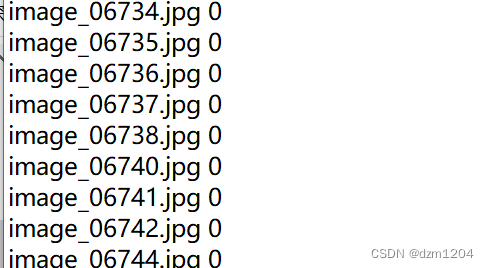### 生成了这样的标签格式后，就需要再写一个数据处理文件，位置在mmcls/datasets下新建一个文件，这里是my_filelist.py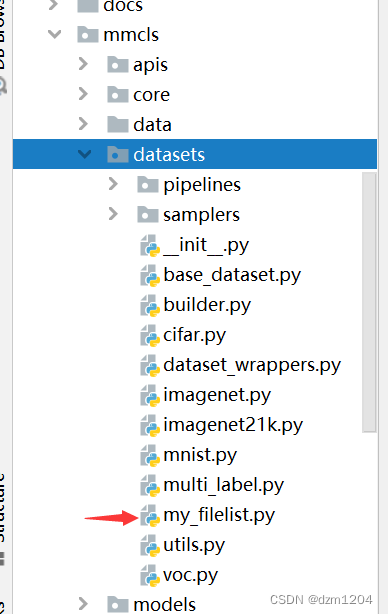### 这里仿照imagenet.py，主要重写下面这个类，写入自己的类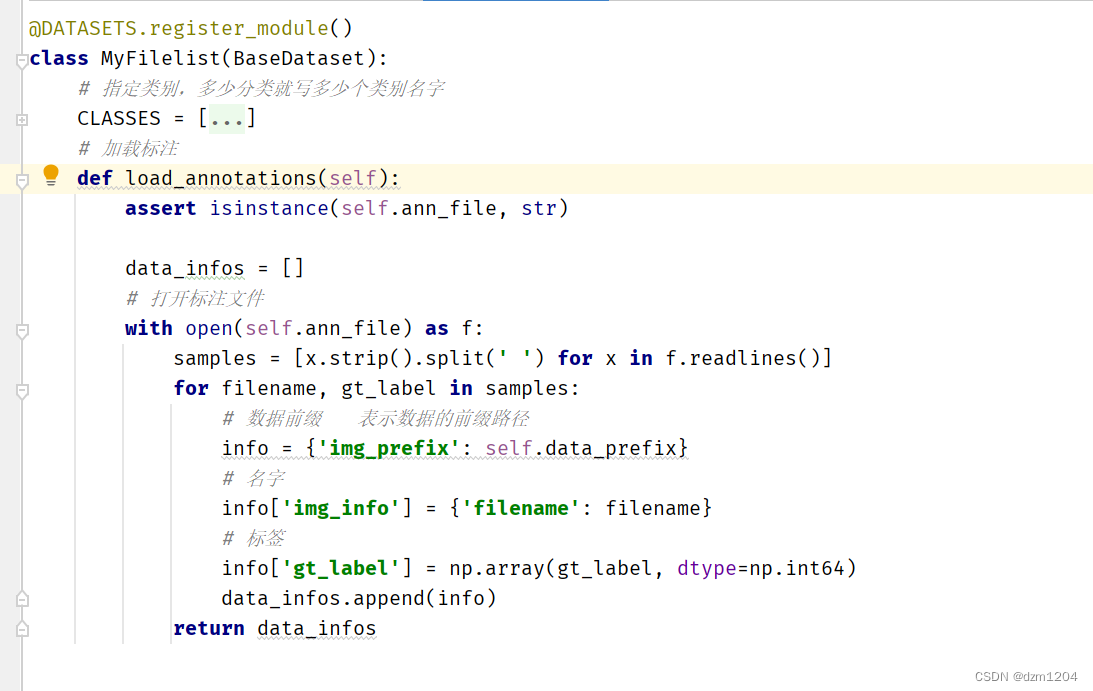### 下一步需要在_init_.py导入自己刚刚写的类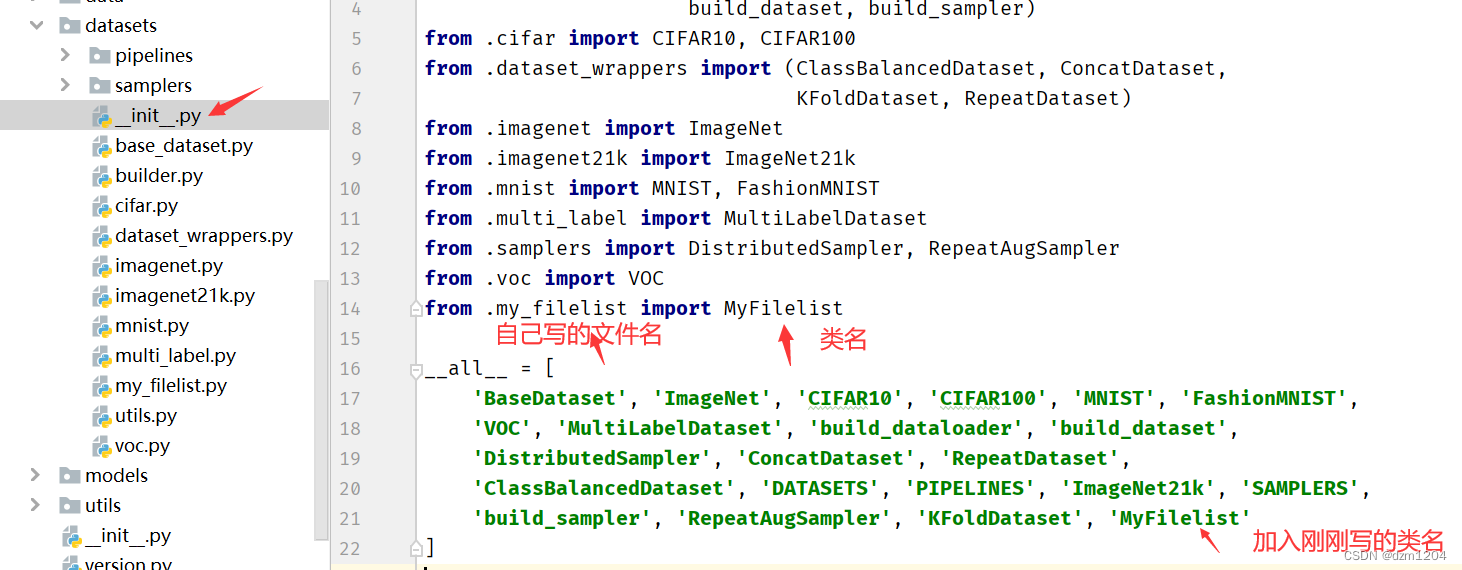### 再去配置文件中去改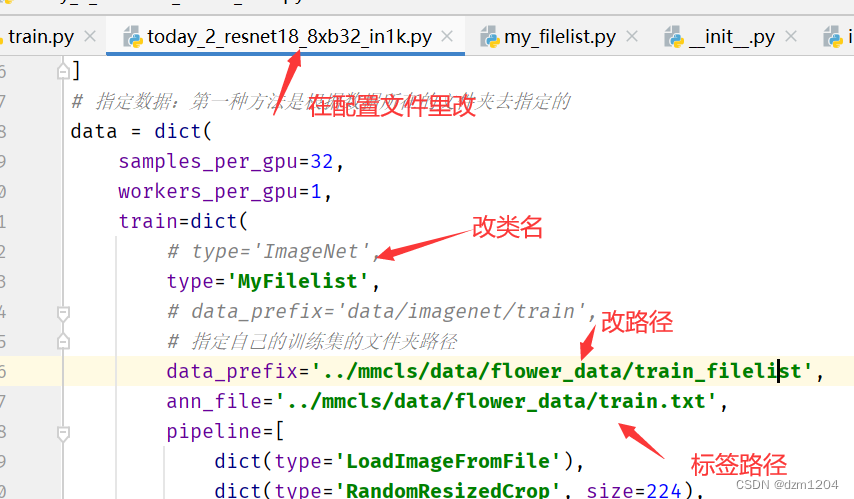### val做同样修改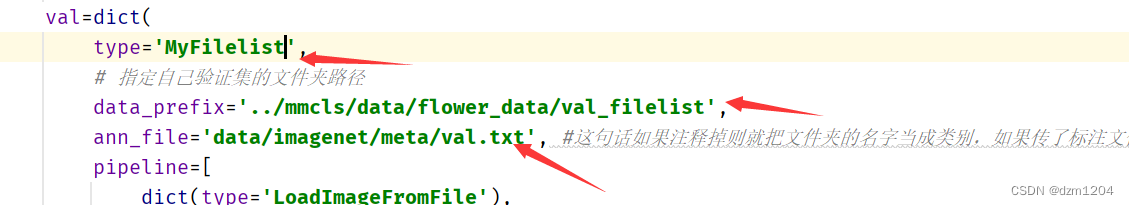### 修改后就可以开始跑自己的任务了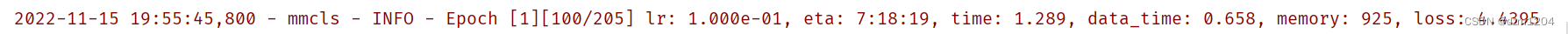### actionSupport类-程序员宅基地

actionSupport类该类实现了action接口和其他的几个有用的接口,比如数据校验、错误消息本地化等.继承该类后,这些功能便自动获得.一、基本校验public void validate(){ PortfolioService ps = getPortfolioService(); if ( getPassword().length() == 0 ){ ..._actionsupport类

### 相对熵(KL散度)-程序员宅基地

https://zhuanlan.zhihu.com/p/37452654https://blog.csdn.net/weixinhum/article/details/85064685交叉熵和相对熵相对熵(KL散度)KL 散度：衡量每个近似分布与真实分布之间匹配程度的方法：\[D_{K L}(p \| q)=\sum_{i=1}^{N} p\left(x_{i}\right)..._为什么相对熵是inf

### 【前端】前端监控体系-程序员宅基地

>对于一个应用来说，除了前期的开发和设计，在项目上线后端维护很重要，其中就包括监控体系的搭建。>系统需要具备发布灰度过程中的监控以及用户问题的反馈和定位等能力。>这些问题可以从2个点解决：数据采集 和 数据上报与监控_前端监控

### TensorFlow2.0教程-AutoGraph-程序员宅基地

TensorFlow2.0教程-AutoGraphtf.function的一个很酷的新功能是AutoGraph，它允许使用自然的Python语法编写图形代码。from __future__ import absolute_import, division, print_functionimport numpy as npimport tensorflow as tffrom tensor...